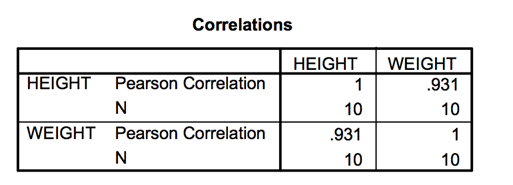# Scatter plot and Correlation Coefficient Assignment Help

## Scatterplot

The ideal way to depict the relationship between two quantitative variables is via Scatterplot It is also known as scatter graph, or scatter diagram.Measured on the same individuals, it portrays the relation between two variables. Here the axes of a graph are use. Cartesian coordinates are plotted to display values in a data set. One variable is depicted on the vertical axis and the other on the horizontal axis.Values of both the first and second variable for an individual is plotted and depicted as point. They can be colour coded. In most case, we are aware if the nature if the variable (whether its explanatory or response), it best to plot explanatory on the horizontal axis calling it x and the other (response) variable y . This can be depicted in the Example below where we use the height and weight of 10 people.

 Height Weight 158 48 162 57 163 57 170 60 154 45 167 55 177 62 170 65 179 70 179 68

Buy putting in the values in the SPSS software we get the following scatterplot:For the sake of understanding the above scatterplot, we need to analyse the pattern all over. It should be able to reveal the following in the form of a pattern: strength, direction and form.

As opposed to the two-way frequency distribution, when “above-average values of one tend to accompany above-average values of the other and below-average values tend to occur together” then both the variables are positively associated. An if above average values of one accompany below average values of the other, they are negatively associated.

What is important is the Linear relationship of both the variables. This occurs when a straight line is formed due to the points plotted. There can be clusters and curves as well. A trend lien which is basically the best fit line is used for both variables to study the relationship between them. This done through linear regression which gives the best solution in time.

Linear Regression is used to understand and model the relationship graphically between a responsive variable and one or more explanatory variables. In bivariate data, simple linear regression is used since there will only be one explanatory variable . This differs from multivariate linear regression.

In the same way, the proximity of the points from each other on the scatterplot can depict the strength of the relationship between the two variables.

### Correlation Coefficient

To understand the strength of the scatterplot quantitatively , we use the correlation coefficient. The scatterplot depicts the relationship between two variables graphically in a data set which is bivariate . Most of the scatterplot cases show points forming around a linear line. This linear relation can be quantified through a measure numerically.

There are many different types of correlation coefficients both of which with different utility and characteristics.

Formally, it can be defined as: “The sample correlation coefficient, denoted by r (or in some cases rxy), is a measure of the strength of the linear relation between the x and y variables ”Height Weight 158162163170154167177170179179 48575760455562657068 -9.9-5.9-4.92.1-13.9-0.99.12.111.111.1 98.0134.8124.014.41193.210.8182.814.41123.21123.21688.9 -10.7-1.7-1.71.3-13.7-3.73.36.311.39.3 114.492.892.891.69187.6913.6910.8939.69127.6986.49588.1 105.9310.038.332.73190.433.3330.0313.23125.43103.23592.7

This will result in the correlation coefficient being:

r = 592.7/ (sq. root of 688.9 x sq. root of 588.1) =  0.9311749

To find yield in SPSS between weight and height, we get the following table :### A correlation coefficient has a number of features that are important :

If the association is positive amongst the variables , then it will results in a positive correlation coefficient , it if it is negative, then it’ll show and negative correlation coefficient .

The value of correlation coefficient is always between -1 and 1. If the value moves away from zero it indicate the relationship is stronger linearly. Furthermore, no matter how strong the relationship is , the correlation coefficient cannot depict the curved relationships in-between variables but only the linear form.

Moreover, just because two variables have a high correlation and have strong association, it doesn't necessarily imply that they are causally related as well. Due to lurking variables, both the variables will be strongly correlated due to their respected association with other variables. This leads to changes in identification.

This form of Correlation coefficient is also referred to as Pearson correlation coefficient popularly. It’s clear from the above observation that only in a case where both the variables are quantitative can Pearson correlation coefficient be used. They are often defined on certain interval scales. However, in some cases, when the variables are qualitative and belong to the ordinal scale, Spearman Correlation coefficient is used to understand the relationship between two such variables. Re sued to make quantitative measurements . The same way the Intra class correlation is used when units that are organised into categories  a However, just like standard deviation, Correlation Coefficient possesses its own set of problems. The data can be skewed due to drastic outliers and a lot of the times , basic statistical inferences are lead to believe in the causal relationships between individuals rather than just the associative relationship.